Students can Download Maths Chapter 2 Measurements Ex 2.1 Questions and Answers, Notes Pdf, Samacheer Kalvi 7th Maths Book Solutions Guide Pdf helps you to revise the complete Tamilnadu State Board New Syllabus and score more marks in your examinations.

## Tamilnadu Samacheer Kalvi 7th Maths Solutions Term 1 Chapter 2 Measurements Ex 2.1

Question 1.
Find the area and perimeter of the following parallelograms.Solution:
(i) Given base b = 11 cm ; height h = 3 cm
Area of the parallelogram = b × h sq. units = 11 × 3 cm2
= 33 cm2
Also perimeter of a parallelogram = Sum of 4 sides
= 11 cm + 4 cm + 11 cm + 4 cm = 30 cm
Area = 33 cm2; Perimeter = 30 cm.

(ii) Given base b = 7 cm
height h = 10 cm
Area of the parallelogram = b × h sq. units
= 7 × 10 cm2 = 70 cm2
Perimeter = Sum of four sides
= 13 cm + 7 cm + 13 cm + 7 cm = 40 cm
Area = 70 cm2, Perimeter = 40 cm

Question 2.
Find the missing values.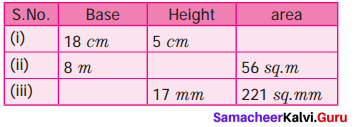Solution:
(i) Given Base 6 = 18 cm ; Height h = 5 cm
Area of the parallelogram = b × h sq. units
= 18 × 5 cm2
= 90 cm2

(ii) Base b = 8m; Area of the parallelogram = 56 sq. m
b × h = 56
8 × A = 56
h = $$\frac{56}{8}$$
h = 7 m

(iii) Given Height h = 17 mm
Area of the parallelogram = 221 sq. mm
b × h = 221
b × 17 = 221
b = $$\frac{221}{17}$$
b = 13 m
Tabulating the results, we get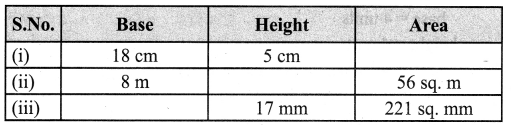Question 3.
Suresh on a parallelogram shaped trophy in a state level chess tournament. He knows that the area of the trophy is 735 sq. cm and its base is 21 cm. What is the height of that trophy?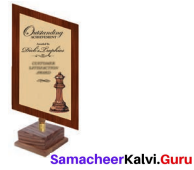Solution:
Given base 6 = 21 cm
Area of parallelogram = 735 sq. cm
b × h = 735
21 × h = 735
h = $$\frac{735}{21}$$
h = 35 cm
∴ Height of the trophy = 35 cmQuestion 4.
Janaki has a piece of fabric in the shape of a parallelogram. Its height is 12 m and its base is 18 m. She cuts the fabric into four equal parallelograms by cutting the parallel sides through its mid-points. Find the area of each new parallelogram.
Solution: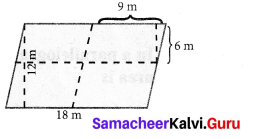Area of a parallelogram = (base × height) sq. units
Base length = $$\frac{18}{2}$$ = 9 m
Height = $$\frac{12}{2}$$ = 6 m
Area = 9 × 6 = 54 m2
Area of each parallelogram = 54 m2

Question 5.
A ground is in the shape of parallelogram. The height of the parallelogram is 14 metres and the corresponding base is 8 metres longer than its height. Find the cost of levelling the ground at the rate of ₹ 15 per sq. m.
Solution: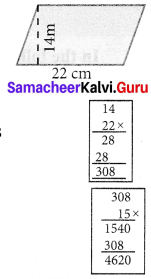Height of the parallelogram h = 14 m
Base = 8 m longer than height
= (14 + 8) m = 22 m
Area of the parallelogram = (base × height) sq. units
= (22 × 14)m2 = 308 m2
Cost of levelling 1 m2 = ₹ 15
Cost of levelling 308 m2 = 308 × 15 = ₹ 4,620
Cost of levelling the ground = ₹ 4,620

Objective Type Questions

Question 6.
The perimeter of a parallelogram whose adjacent sides are 6 cm and 5 cm is
(i) 12 cm
(ii) 10 cm
(iii) 24 cm
(iv) 22 cm
Solution:
(iv) 22 cm
Hint:
= 2(6 + 5) = 2 × 11 = 22 cm

Question 7.
The area of parallelogram whose base 10 m and height 7 m is
(i) 70 sq.m
(ii) 35 sq.m
(iii) 7 sq.m
(iv) 10 sq.m
Solution:
(i) 70 sq. m
Hint: = base × height = 10m × 7m = 70 sq.m

Question 8.
The base of the parallelogram with area is 52 sq. cm and height 4 cm is
(i) 48 cm
(ii) 104 cm
(iii) 13 cm
(iv) 26 cm
Solution:
(iii) 13 cm
Hint: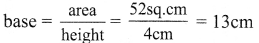Question 9.
What happens to the area of the parallelogram if the base is increased 2 times and the height is halved?
(i) Decreases to half
(ii) Remains the same
(iii) Increase by two times
(iv) None
Solution:
(ii) Remains the same
Hint:
Area = b × h sq. units
New base = 2 × old base
New height = $$\frac{1}{2}$$ × old height
New Area = New base × New height = (2 × b)$$\frac{1}{2}$$ × h = bh = old Area.Question 10.
In a parallelogram the base is three times its height. If the height is 8 cm then the area is
(i) 64 sq. cm
(ii) 192 sq. cm
(iii) 32 sq. cm
(iv) 72 sq. cm
Solution:
(ii) 192 sq. cm
Hint: Given b = 3 × h; h = 8 cm
Area = b × h = 3h × 8 = 3 × 8 × 8 = 192 cm2